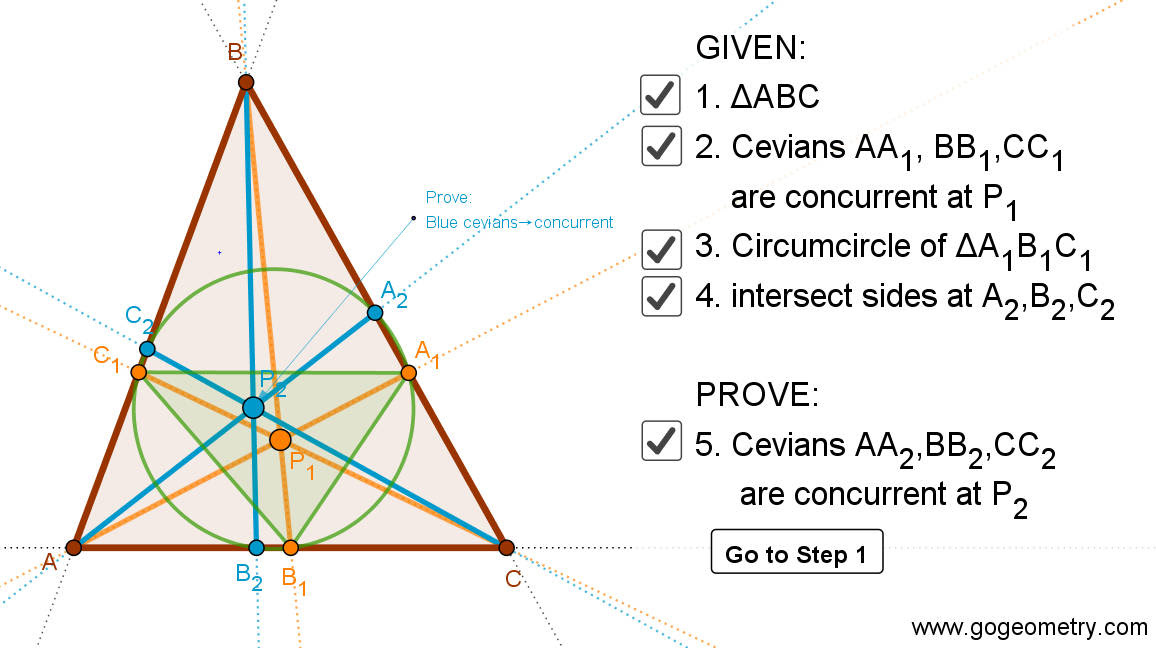## Thursday, May 7, 2020

### Dynamic Geometry 1478: Reuschle-Terquem Theorem, Concurrent Cevians, Triangle, Circumcircle, Secant line, Cyclocevian, Step-by-step Illustration

Interactive step-by-step animation using GeoGebra. Post your solution in the comment box below.
Level: Mathematics Education, High School, Honors Geometry, College.

Details: Click on the figure below.1.Let n=BA1 .BA2 = BC1.BC2
and m=AB1.AB2=AC1.AC2 ; p=CA1.CA2=CB1.CB2
1. Apply Ceva’s theorem for triangle ABC and point of concurrent P1
(A1B/A1C) * (B1C/B1A) *(C1A/C1B)=1

2. Replace BA1=m/BA2 ; CA1=p/CA2 etc…. in above equation and simplify we have :
we get (A2B/A2C) (B2C/B2A)(C2A/C2B)= 1 .
Per Ceva’s theorem AA2,BB2, CC2 are concurrent at P2

2.From Ceva's theorem ( the power of P1 ) we get(AB1/CB1).(CA1/BA1).(BC1/AC1) = 1
Substitute BA1/BC1 = BC2/BA2 and so on ... => (AB2/CB2).(CA2/BA2).(BC2/AC2) = 1